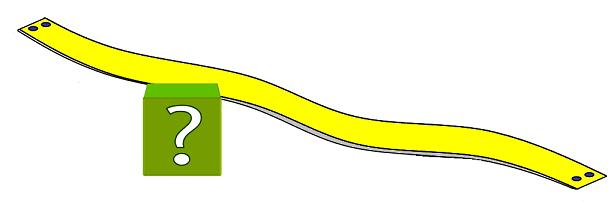#### You may also like### Euler's Squares

Euler found four whole numbers such that the sum of any two of the numbers is a perfect square...### Odd Differences

The diagram illustrates the formula: 1 + 3 + 5 + ... + (2n - 1) = nÂ² Use the diagram to show that any odd number is the difference of two squares.### Tree Tops

Can you make sense of information about trees in order to maximise the profits of a forestry company?

# Under the Ribbon

##### Age 14 to 16Challenge Level

A ribbon is nailed down with a small amount of slack.
The distance between the nailed ends is 1 metre and the amount of ribbon is 104 cm.

What is the largest cube that can pass under the ribbon ?

And where along the ribbon is the best place for the cube to pass under, or doesn't the position matter ?